# How to Reverse a Linked List

## How to Reverse a Linked List in C Language:

In this article, I am going to discuss How to Reverse a Linked List in C Language with Examples. Please read our previous article, where we discussed How to Remove Duplicates from Linked List in C Language with Examples.

##### How to Reverse a Linked List:

Here, we will learn how to reverse a linked list. Reversing a linked list means we have to change the order of the elements. Let us understand this with an example.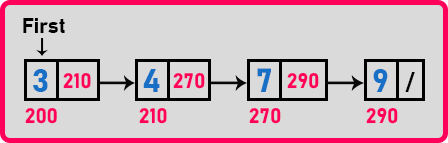In this linked list, the order of elements is 3, 4, 7, and 9. Then we have to reverse the order as 9, 7, 4, and 3. There are two methods for reversing a linked list:

1. Reversing Elements of a Linked List

In reversing elements method, the value of the 1st node is ‘3’ and the last node is ‘9’, so we should interchange them. The node will remain the same but the data will interchange.

In reversing links method, the order of nodes is ‘200 -> 210 -> 270 -> 290’. But we have to reverse the links so the order becomes ‘200 <- 210 <- 270 <- 290’. So, the value remains in the same node. Only the links will change and the first node becomes the last node and the last node becomes the first node i.e. before reverse- ‘3’ is the first node and ‘9’ is the last node, after reverse- ‘3’ becomes the last node and ‘9’ will be the first node. So, in this method, we prefer reversing links rather than reversing elements.

These are the two approaches. One is reversing elements and another is reversing links. Let us look at the first method which is reversing the elements.

##### Reversing Elements of a Linked List:We can take an array of the same size as the number of elements in the linked list. In this linked list there are four elements, so we should take an array of size ‘4’.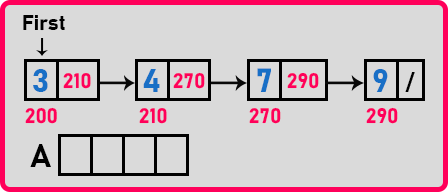Then we should copy all these elements from the linked list to the array.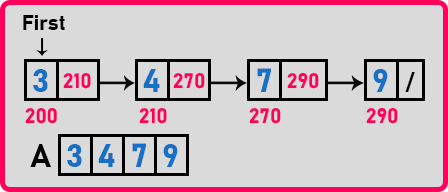After copying all the elements, then again reverse copy all the elements in that list.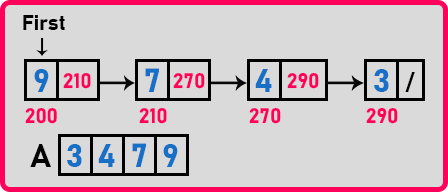Now the elements are in reverse order in the linked list. By taking an auxiliary array we can reverse elements in a linked list. Let us write pseudo code for this method.

##### Pseudo Code for Reversing Elements of a Linked List:

p = first;
i = 0;
while(p != NULL){
A[i] = p->data;
p = p->next;
i++;
}
p = first; i–;
while(p != Null){
p->data = A[i–];
p = p->next;
}

Now let us write the complete program.

##### Program for Reversing Elements of a Linked List using C Language:
```#include <stdio.h>
#include <stdlib.h>

struct Node
{
int data;
struct Node *next;
} *first = NULL;

void Create(int A[], int n)
{
int i;
struct Node *t, *last;
first = (struct Node *) malloc (sizeof (struct Node));
first->data = A;
first->next = NULL;
last = first;

for (i = 1; i < n; i++)
{
t = (struct Node *) malloc (sizeof (struct Node));
t->data = A[i];
t->next = NULL;
last->next = t;
last = t;
}
}

int Count(struct Node * p) {
int l = 0;
while (p) {
l++;
p = p -> next;
}
return l;
}

void Display(struct Node *p)
{
while (p != NULL)
{
printf ("%d ", p->data);
p = p->next;
}
}

void ReverseElements(struct Node *p)
{
int *A, i = 0;
struct Node *q = p;
A = (int *) malloc(sizeof(int) * Count(p));

while (q != NULL)
{
A[i] = q->data;
q = q->next;
i++;
}

q = p;
i--;
while (q != NULL)
{
q->data = A[i];
q = q->next;
i--;
}
}

int main()
{
int A[] = { 3, 4, 9, 10, 13, 16, 19 };
Create(A, 5);

printf("Before Reverse: \n");
Display(first);

ReverseElements(first);

printf("\nAfter Reverse: \n");
Display(first);
return 0;
}
```
###### Output: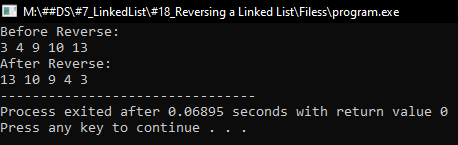In the next article, I am going to discuss How to Reverse a Linked List by Reversing Links in C Language with Examples. Here, in this article, I try to explain How to Reverse a Linked List in C Language with Examples and I hope you enjoy this Reversing a Linked List in C Language with Examples article.